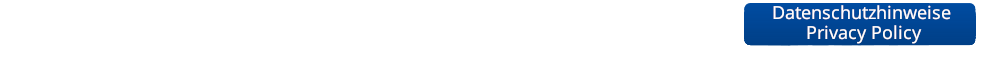Advanced Machine Learning

 Semester: WS 2012 Type: Lecture Lecturer: Credits: None

This lecture will extend the scope of the "Machine Learning" lecture with additional and, in parts, more advanced concepts. In particular, the lecture will cover the following areas:

• Regression techniques (linear regression, ridge regression, lasso, support vector regression)
• Gaussian Processes
• Learning with latent variables
• Dirichlet Processes
• Structured output learning
Course Schedule
Date Title Content Material
Introduction Introduction, Polynomial Fitting, Least-Squares Regression, Overfitting, Regularization, Ridge Regression
Linear Regression I Probabilistic View of Regression, Maximum Likelihood, MAP, Bayesian Curve Fitting
Linear Regression II Basis Functions, Sequential Learning, Multiple Outputs, Regularization, Lasso, Bias-Variance Decomposition
Gaussian Processes I Kernels, Kernel Ridge Regression, Gaussian Processes, Predictions with noisy observations
Gaussian Processes II Influence of hyperparameters, Bayesian Model Selection, GP Classification
Probability Distributions Bayesian Estimation Revisited, Conjugate Priors, Bernoulli, Binomial, Beta, Multinomial, Dirichlet, Gaussian, Gamma, Student's t
Approximate Inference I Sampling Approaches, Monte Carlo Methods, Transformation Methods, Ancestral Sampling, Rejection Sampling, Importance Sampling
Approximate Inference II Markov Chain Monte Carlo, Metropolis-Hastings Algorithm, Gibbs Sampling
Mixture Models I Mixtures of Gaussians, ML estimation, EM, latent variables, General EM
Mixture Models II General EM, Mixtures of Bernoulli distributions, Monte Carlo EM, Dirichlet priors, Infinite Mixture Models, Gibbs Sampling
Dirichlet Processes Definition, Properties, Polya Urn scheme, Chinese Restaurant Process
Dirichlet Processes II De Finetti's Theorem, Exchangeability, Stick-Breaking Construction, DP Mixture Models
Hierarchical DPs Gibbs Sampling for DPMMs, Hierarchical DPs, Chinese Restaurant Franchise
Hierarchical DPs II Gibbs Sampling for HDPs, Applications
Latent Factor Models Latent Factor Models, PCA, Probabilistic PCA, ML for PCA, Factor Analysis, ICA, Sparse Latent Factor Models
Beta Processes I Finite Latent Factor Models, Infinite Latent Factor Models, Indian Buffet Process
Beta Processes II Beta Processes, Stick-Breaking Construction, Gibbs Sampling for BPs, Applications, Nonparametric HMMs
Support Vector Machines SVMs revisited, Loss functions
SV Regression Kernel PCA, Kernel k-Means, Support Vector Data Description, Support Vector Regression
Structured Output Learning General structured prediction, Loss functions, Structured Output SVM, Cutting plane training
Structured Output Learning II One-Slack formulation, Multi-class SVM, Joint kernel function, Kernelized S-SVM